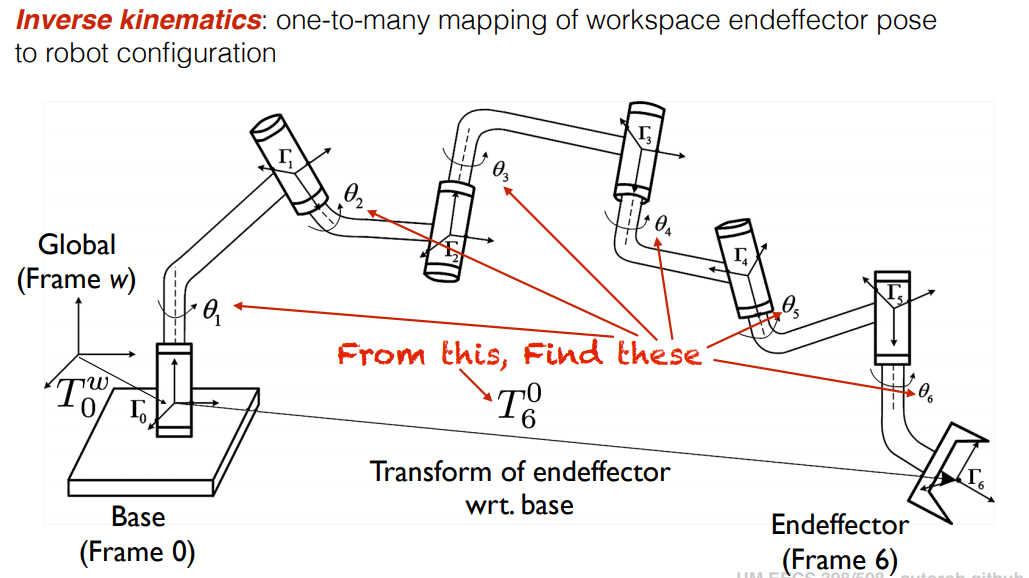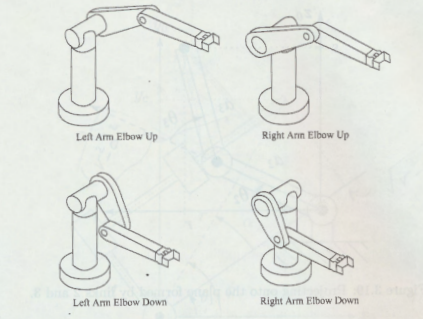Inverse Kinematics

Inverse Kinematics

In robotics, inverse kinematics makes use of the kinematics equations to determine the joint parameters that provide a desired position for each of the robot's end-effectors. Specification of the movement of a robot so that its end-effectors achieve the desired tasks is known as motion planning. Inverse kinematics transforms the motion plan into joint actuator trajectories for the robot.

In short you can think about it this way, if we know the end position of the robot, we are also able to decide how the joints and links needs to be positioned in order for the manipulator to result in that end position.Analytical solutions

An analytic solution to an inverse kinematics problem is a closed-form expression that takes the end-effector pose as input and gives joint positions as output, q=f(x). Analytical inverse kinematics solvers can be significantly faster than numerical solvers and provide more than one solution for a given end-effector pose.

It's important to see why we find multiple rotational and translational answers for one trajectory for the manipulator. You can imagine this for example, extend your arm to an object, now you have one way of manipulating your arm to that object. Now rotate your elbow by 90 degrees, and voila, you have now one more answer to the problem.

Here's an image showing 4 possible answers for a manipulator, knowing only the end-effectorHaving multiple answers to one problem is very useful for robotics since we may need to eliminate some of the answers according to the obstacles or the terrain we are working on.